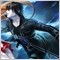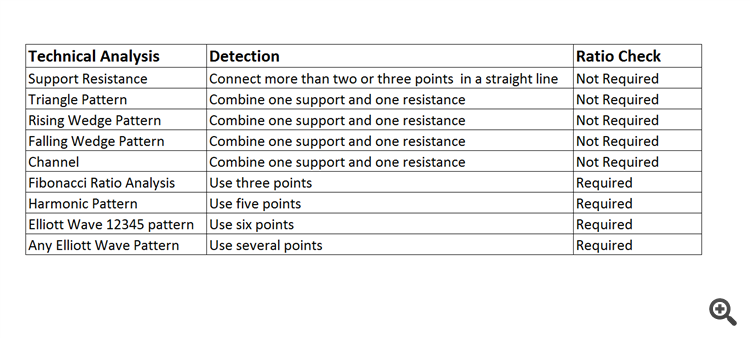# Fractal Wave For Forex Trading (and Stock Market Trading) - page 298912

Here is the summary of the techncial analysis in the table format assuming the peak trough analysis is applied to your chart. The points means either peak or trough here. For example, "Connect more than two or three points in a straight line" means to connect more than two or three peaks in a straight line (=Resistance) or to connect more than two or three troughs in a straight line (=Support).Some technical analysis do not require ratio check and some require the ratio check.

Ratio check simply means to divide the price change of the right swing by the price change of the left swing in the fractal triangle (see the screenshot below).

Ratio = Y0/Y1.

That is it. To understand the technical anlaysis, you do not need to understand some rocket science but just understanding simple ratio like this is more than sufficient.

Hence, anyone can attempt to learn and to practically use them for their trading.

I wrote this information in a hope that you can find some consistency across all the techncal analysis. This will help you to understand any techncial analysis with confidence in the future.

When I first tried to learn any of these techniques many years ago, no one provided some clear explanation on how each techniques are related together.

Hence, at that time, I had to spend a lot of time to learn each technique seperately. Sometimes, this could be the bottle neck of your Forex education.

But as you can see, all these techniques are just different language to describe (hence, to predict) the fractal wave present in the forex and stock market.

If you try to understand fractal geometry of forex market first, then the learing time for all these technical analysis can be shorten dramatically.

You can also set much clearer objective for your techncial analysis too.